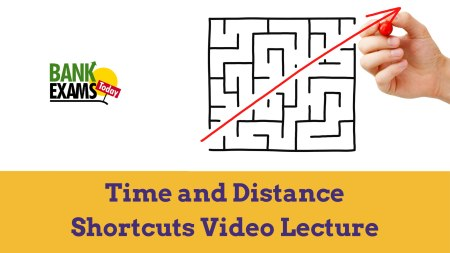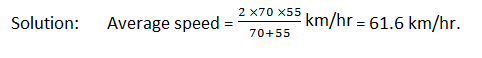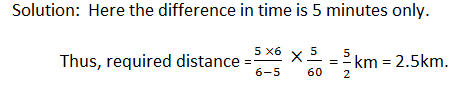New Students Offer - Use Code HELLO

# Time and Distance - Concept and Questions {Video Lecture}Time and Distance is an important chapter from examination point of view.

### FORMULAE:

1. Speed = Distance/Time
2. Time = Distance/Speed
3. Distance = Speed × Time
4. If the speed of a body is changed in the ratio a : b, then the ratio of the time taken changes in the ratio b : a.
5. m km/hr = [m × 5/18] m/sec.
6. m metres/sec = [m × 18/5] km/hr.
I recommend you to watch the following concept video before solving the questions.

#### Question 1.

Express a speed of 18 km/hr in metres per second.

Solution: 18 km/hr = [18 × 5/18] m/sec. = 5 metres/sec.

#### Question 2.

Express 10 m/sec. in km/hr.
Solution: 10 metres/sec = [10 × 18/5] km/hr. = 36 km/hr.

#### THEOREM

If a certain distance is covered at m km/hr and the same distance is covered at n km/hr then the average speed during the whole journey is 2mn/(m+n) km/hr.

Let the distance be A km.

Time taken to travel the distance at a speed of m km/hr = A/m hrs.
Time taken to travel the distance at a speed of n km/hr = A/n hrs.
we see that the total distance of 2A km is travelled in (A)/m+A/n hrs.#### Question 3.

Rakesh covers a certain distance by car driving at 70 km/hr and he returns to the starting point riding on a scooter at 55 km/hr. Find his average speed for the whole journey.#### Question 4.

Raju covers distance between his house and office on scooter. Having an average speed of 30 km/hr, he is late by 10 min. However, with a speed of 40 km/hr, he reaches his office 5 min earlier. Find the distance between his house and office.#### Question 5.

A man walking with a speed of 5 km/hr reaches his target 5 minutes late. If he walks at a speed at a speed of 6 km/hr, he reaches on time. Find the distance of his target from his house.#### Question 6.

A boy goes to school at a speed of 3 km/hr and returns to the village at a of 2 km/hr. if he takes 5 hrs in all, what is the distance between the village and the school?